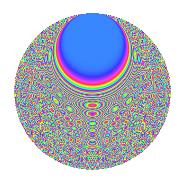# Properties

 Label 8009.2.aLevel 8009 Weight 2 Character orbit a Rep. character $$\chi_{8009}(1,\cdot)$$ Character field $$\Q$$ Dimension 667 Newform subspaces 2 Sturm bound 1335 Trace bound 1

# Related objects

## Defining parameters

 Level: $$N$$ $$=$$ $$8009$$ Weight: $$k$$ $$=$$ $$2$$ Character orbit: $$[\chi]$$ $$=$$ 8009.a (trivial) Character field: $$\Q$$ Newform subspaces: $$2$$ Sturm bound: $$1335$$ Trace bound: $$1$$

## Dimensions

The following table gives the dimensions of various subspaces of $$M_{2}(\Gamma_0(8009))$$.

Total New Old
Modular forms 668 668 0
Cusp forms 667 667 0
Eisenstein series 1 1 0

The following table gives the dimensions of the cuspidal new subspaces with specified eigenvalues for the Atkin-Lehner operators and the Fricke involution.

$$8009$$Dim.
$$+$$$$306$$
$$-$$$$361$$

## Trace form

 $$667q - 3q^{2} - 2q^{3} + 667q^{4} - 4q^{5} + 4q^{7} - 3q^{8} + 657q^{9} + O(q^{10})$$ $$667q - 3q^{2} - 2q^{3} + 667q^{4} - 4q^{5} + 4q^{7} - 3q^{8} + 657q^{9} + 4q^{10} - 10q^{11} + 2q^{12} - 8q^{14} - 6q^{15} + 663q^{16} - 10q^{17} - 23q^{18} + 6q^{19} - 18q^{20} - 10q^{21} + 6q^{22} - 10q^{23} + 8q^{24} + 667q^{25} - 8q^{27} + 12q^{28} - 20q^{29} - 36q^{30} + 14q^{31} - 9q^{32} - 12q^{33} - 6q^{34} - 6q^{35} + 625q^{36} - 6q^{37} - 20q^{38} + 20q^{39} + 26q^{40} - 18q^{41} + 12q^{42} + 10q^{43} - 24q^{44} - 18q^{45} + 10q^{46} + 12q^{47} - 8q^{48} + 679q^{49} - 13q^{50} - 2q^{51} + 4q^{52} - 34q^{53} + 8q^{54} + 32q^{55} - 64q^{56} - 14q^{57} + 12q^{58} - 14q^{59} - 34q^{60} - 6q^{61} + 40q^{63} + 707q^{64} - 30q^{65} - 22q^{66} + 12q^{67} + 40q^{68} - 28q^{69} + 32q^{70} - 26q^{71} - 39q^{72} - 10q^{73} - 6q^{74} + 16q^{75} + 72q^{76} - 8q^{77} + 22q^{78} + 18q^{79} - 60q^{80} + 587q^{81} + 44q^{82} + 38q^{83} - 68q^{84} + 4q^{85} - 52q^{86} + 26q^{87} + 14q^{88} - 46q^{89} - 78q^{90} + 16q^{91} - 86q^{92} - 28q^{93} + 24q^{94} + 28q^{95} + 32q^{96} + 8q^{97} + 21q^{98} - 62q^{99} + O(q^{100})$$

## Decomposition of $$S_{2}^{\mathrm{new}}(\Gamma_0(8009))$$ into newform subspaces

Label Dim. $$A$$ Field CM Traces A-L signs $q$-expansion
$$a_2$$ $$a_3$$ $$a_5$$ $$a_7$$ 8009
8009.2.a.a $$306$$ $$63.952$$ None $$-13$$ $$-25$$ $$-25$$ $$-102$$ $$+$$
8009.2.a.b $$361$$ $$63.952$$ None $$10$$ $$23$$ $$21$$ $$106$$ $$-$$

## Hecke characteristic polynomials

There are no characteristic polynomials of Hecke operators in the database